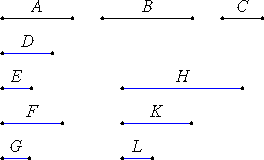# Proposition 12

Magnitudes commensurable with the same magnitude are also commensurable with one another.

Let each of the magnitudes A and B be commensurable with C.

I say that A is also commensurable with B.X.5

Since A is commensurable with C, therefore A has to C the ratio which a number has to a number. Let it have the ratio which D has to E. Again, since C is commensurable with B, therefore C has to B the ratio which a number has to a number. Let it have the ratio which F has to G.

VIII.4

And, given any number of ratios we please, namely the ratio which D has to E and that which F has to G, take the numbers H, K, and L continuously in the given ratios, so that D is to E as H is to K, and F is to G as K is to L.

V.11

Since A is to C as D is to E, while D is to E as H is to K, therefore A is to C as H is to K. Again, since C is to B as F is to G, while F is to G as K is to L, therefore C is to B as K is to L.

V.22

But A is to C as H is to K, therefore, ex aequali, A is to B as H is to L.

X.6

Therefore A has to B the ratio which a number has to a number. Therefore A is commensurable with B.

Therefore, magnitudes commensurable with the same magnitude are also commensurable with one another.

Q.E.D.

## Guide

The proof is primarily an application of VIII.4.

This proposition is used in frequently in Book X starting with the next proposition. It is also used in XIII.11.